# Align Slice Modifier gizmo to 3points

Hello everyone,

I have a simple problem. I need quickly and easily tool to align slice gizmo by three points. Is there anyone who help me please?

Thanks so muchMira

situation 1)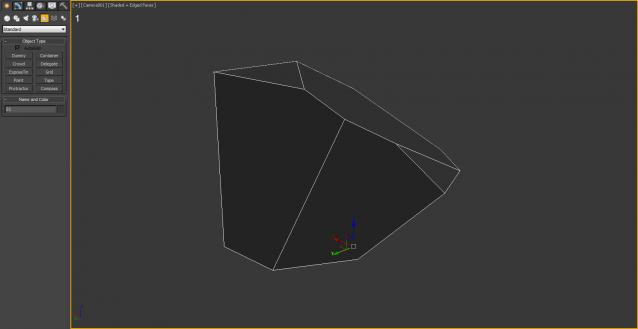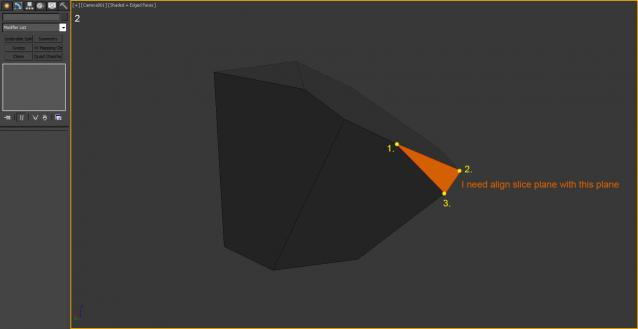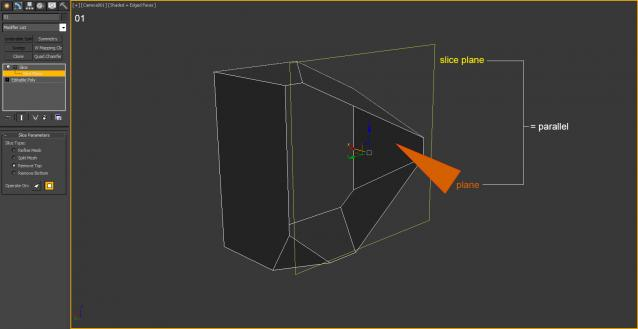situation 2)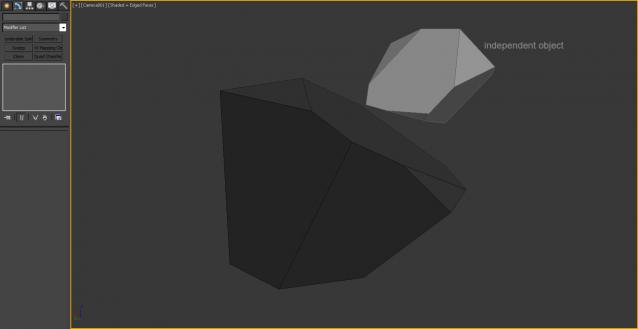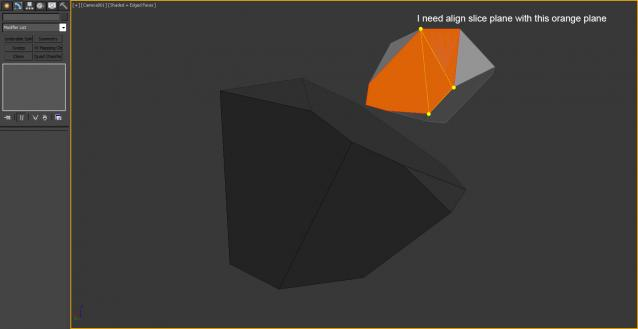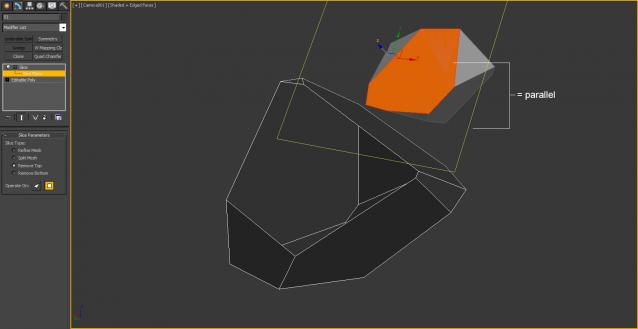## Comment viewing options

Select your preferred way to display the comments and click "Save settings" to activate your changes.### in perspective viewport move

in perspective viewport move and rotate the as you wish and use quick slice tool in editpoly.### .

```(
curO = selection
sliceMod = curO.modifiers
--	get the three points and builda matrix
pos01 = polyop.getVert curO 8
pos02 = polyop.getVert curO 5
pos03 = polyop.getVert curO 2
--
local vect1 = normalize (pos02 - pos01)
local vect2 = normalize (pos03 - pos02)
local vect3 = normalize (cross vect2 vect1)
vect2 = cross vect3 vect1
--
objTM = curO.objectTransform
modTM = getModContextTM curO sliceMod
-- set slice plane position at world coordinates f one of the points
pos = ((pos01 + pos02 + pos03) / 3) * modTM * (inverse objTM)
--	build temp matrix
tempMatrix = ((matrix3 vect1 vect2 vect3 pos ))
--	align the slice plane
curO.slice.slice_plane.transform = tempMatrix
)```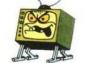### Hi Kostadin, thank you very

thank you very much for your help.
Cube was a example. I need select the points anywhere with any object. I update my post – new image
There is good script but dont work with gizmo http://www.scriptspot.com/3ds-max/scripts/3-point-align

mira### .

Try this:

```(
on execute do  with undo off
(
curO = selection
if curO.modifiers.count != 0 and classOf (sliceMod = curO.modifiers) == SliceModifier do
(
local pos01 = undefined
local pos02 = undefined
local pos03 = undefined

local curSnapMode = snapMode.active
local curSnapType = snapMode.type
snapMode.active = true
snapMode.type = #3D

pos01 = pickPoint snap:#3d
if classof pos01 == point3 do
pos02 = pickPoint snap:#3d rubberBand:pos01
if classof pos02 == point3 do
pos03 = pickPoint snap:#3d rubberBand:pos02

snapMode.active = curSnapMode
try(snapMode.type = curSnapType)catch()
if classof pos01 == point3 and classof pos02 == point3 and classof pos03 == point3 do
(				--
local vect1 = normalize (pos02 - pos01)
local vect2 = normalize (pos03 - pos02)
local vect3 = normalize (cross vect2 vect1)
vect2 = cross vect3 vect1
--
objTM = curO.objectTransform
modTM = getModContextTM curO sliceMod
-- set slice plane position at world coordinates f one of the points
pos = ((pos01 + pos02 + pos03) / 3) * modTM * (inverse objTM)
--	build temp matrix
tempMatrix = ((matrix3 vect1 vect2 vect3 pos ))
--	align the slice plane
curO.slice.slice_plane.transform = tempMatrix
)
)
)
)```

Run it and pick 3 points in the scene. The SNAP will be turned On automatically.### .

Inspired from this one:
http://www.scriptspot.com/forums/3ds-max/general-scripting/slice-script

Would you please do a modification of the code so that it adds a new modifier instead of affecting the existing?

Kind regards
Haider
www.haider.se### .

```(
on execute do  with undo off
(
curO = selection
sliceMod = SliceModifier ()
local pos01 = undefined
local pos02 = undefined
local pos03 = undefined

local curSnapMode = snapMode.active
local curSnapType = snapMode.type
snapMode.active = true
snapMode.type = #3D

pos01 = pickPoint snap:#3d
if classof pos01 == point3 do
pos02 = pickPoint snap:#3d rubberBand:pos01
if classof pos02 == point3 do
pos03 = pickPoint snap:#3d rubberBand:pos02

snapMode.active = curSnapMode
try(snapMode.type = curSnapType)catch()
if classof pos01 == point3 and classof pos02 == point3 and classof pos03 == point3 do
(				--
local vect1 = normalize (pos02 - pos01)
local vect2 = normalize (pos03 - pos02)
local vect3 = normalize (cross vect2 vect1)
vect2 = cross vect3 vect1
--
objTM = curO.objectTransform
modTM = getModContextTM curO sliceMod
-- set slice plane position at world coordinates f one of the points
pos = ((pos01 + pos02 + pos03) / 3) * modTM * (inverse objTM)
--	build temp matrix
tempMatrix = ((matrix3 vect1 vect2 vect3 pos ))
--	align the slice plane
curO.slice.slice_plane.transform = tempMatrix
)
)
)```### .

thanks a lot :)

Kind regards
Haider
www.haider.se### .

It is a great script request though. It makes sense, wanting to align the gizmo in a certain way. It could as well being the UV gizmo that you want to align to those three verts..

Kind regards
Haider
www.haider.se### not tool but suggestion...

Assumming your object is far more complexed than a simple cube,
what about make 3 cuts, ie. cut from vert 1 to 2 to 3, then select the 'other' vert at the tip, grow until desired, then delete...?

## Comment viewing options

Select your preferred way to display the comments and click "Save settings" to activate your changes.